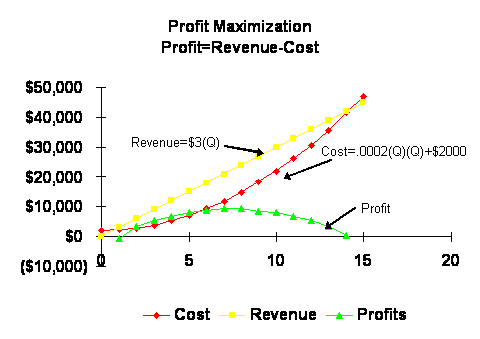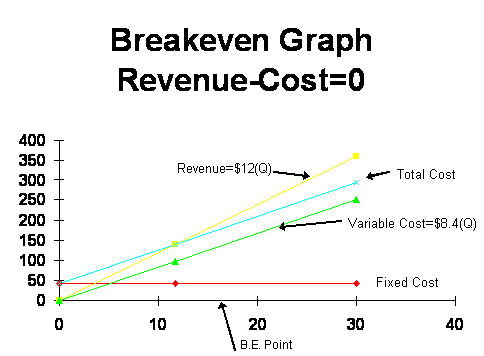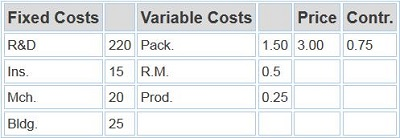# Marketing Notes – Quantitative Methods Review

Basic notes from core MBA marketing course, focusing on quantitative methods review
Subject: Marketing

## Performance Maximization – Optimal Sales Goal

Given the following, how do we maximize performance?
Revenue per unit is \$3.00
Fixed costs are \$2000
Variable cost of \$.0002 times Quantity2### Use of the Calculus

• Unconstrained problem in a single variable:
• Maximize P = 3Q – 0.0002Q2 – 2000

• Set first derivative equal to 0 to obtain Q* = 7500 and P* = 9250
• Second derivative is < 0, so the profit function is concave and Q* corresponds to a maximum

If the budget is \$10,000, what are the optimal allocations for advertising and promotion?
A sales manager estimates that monthly sales can be represented by

S = 60,000 + 3A – 0.00025A2 + 6P – 0.004P2

Where:
S = sales in units
P = promotion activity in dollars

If the budget is \$10,000, what are the optimal allocations for advertising and promotion?

## Constrained Optimization

Constrained problem in multiple variables:
Max S = 60,000 + 3A – 0.00025A2 + 6P – 0.004P2
s.t. A + P = 10,000

Use of Lagrange Multipliers
Optimal solution:
A* = \$5923, P* = \$4077, S* = 26,973
Sales function is concave so we have a maximum.

## Breakeven Analysis

The prime condition is:
Revenue = Total Cost
Price * Quantity = Fixed Cost + (Variable Cost) * Quantity
BEQ = FC ÷ (price – VC)

## Important Breakeven Concepts

• Fixed Cost
• Variable Cost
• Marginal Cost
• Relevant Cost
• Sunk Cost
• Marginal Revenue
• Price
• Contribution (price – VC)

Example:
Total Cost = \$42,000 + \$8.4 * Q
Revenue = \$12 * Q
Breakeven condition:
Revenue – Total Cost = 0
\$12 * Q – \$42,000 – \$8.4 * Q = 0
BEQ = 11,667 unitsExample:
Your department is “brainstorming” for ideas to meet earnings goals imposed by higher management. One idea sounds good, but you remember the corporate planning staff reviews your ideas using terms like net present value, contribution, etc.

Available Information

• R&D: \$220,000
• Raw materials/unit: \$0.50
• Production cost/unit: \$0.25
• Insurance: \$15,000
• Building: \$25,000
• Machinery: \$20,000
• Packaging: \$1.50
• Selling Price: \$3.00

How many units must be sold to break even?

Solution:BEQ = FC ÷ Contribution = 280,000 ÷ 0.75 = 373,334

Including a Target:

• Firm requires an IRR of 25% on its investments
• How many units must be sold to reach the firm’s requirement?

Solution:

BEQ = (FC + Target) ÷ Contribution
= (FC + 0.25(FC)) ÷ 0.75
= (280,000 * 1.25) ÷ 0.75 = 466,667

## Including an After Tax Target:

• You note your firm requires an after tax return of 25% on its investments.
• How many units must be sold to reach the firm’s requirement?

Solution:
BEQ = (FC + After Tax Target) ÷ Contribution
= (FC + (0.25*FC) ÷ (1 – MTR)) ÷ 0.75
= (280,000 (1 + 0.25 ÷ 0.6)) ÷ 0.75
= 529,000

## Which Approach is Better?

• Optimization methods
• identify a specific maximum
• require extensive information
• require high level skills
• Contribution method is
• Simple
• relatively easy
• sufficiently accurate given incomplete information

There Is 1 Comment